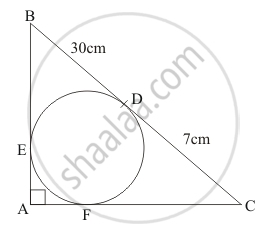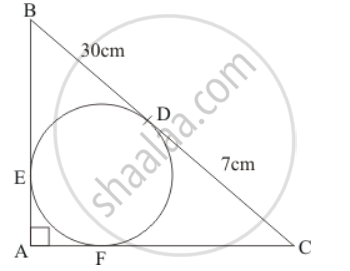Advertisement Remove all ads

# In the Given Figure, Bdc is a Tangent to the Given Circle at Point D Such that Bd = 30 Cm and Cd = 7 Cm.(I) Af - Mathematics

Short Note

In the given figure, BDC is a tangent to the given circle at point D such that BD = 30 cm and CD = 7 cm. The other tangents BE and CF are drawn respectively from B and C to the circle and meet when produced at A making BAC a right angle triangle. Calculate (i) AFAdvertisement Remove all ads

#### Solution

The given figure is below(i) The given triangle ABC is a right triangle where side BC is the hypotenuse. Let us now apply Pythagoras theorem. We have,

AB^2+AC^2=BC^2

Looking at the figure we can rewrite the above equation as follows.

(BE+EA)^2+(AF+FC)^2=(30+7)^2 …… (1)

From the property of tangents we know that the length of two tangents drawn from the same external point will be equal. Therefore we have the following,

BE = BD

It is given that BD = 30 cm. Therefore,

BE = 30 cm

Similarly,

CD = FC

It is given that CD = 7 cm. Therefore,

FC = 7 cm

Also, on the same lines,

EA = AF

Let us substitute these in equation (1). We get,

(BE+EA)^2+(AF+FC)^2=(30+7)^2

(30+AF)^2+(AF+7)^2=37^2

(30^2+2 xxAF+AF^2)+(AF^2+2xx7xxAF+7^2)=1369

900+60AF+AF^2+AF^2+14AF+49=1369

2F^2+74AF-420=0

AF^2+37AF-210=0

AF^2(AF+42)-5(AF+42)=0

AF(AF+42)-5(AF+42)=0

(AF-5)(AF+42)=0

Therefore,

Therefore,

AF = 5

Or,

AF = − 42

Since length cannot have a negative value,

AF = 5

Is there an error in this question or solution?
Advertisement Remove all ads

#### APPEARS IN

RD Sharma Class 10 Maths
Chapter 8 Circles
Exercise 8.2 | Q 29.1 | Page 37
Advertisement Remove all ads
Advertisement Remove all ads
Share
Notifications

View all notifications

Forgot password?